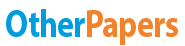# Cpr 1: Titration

Essay by   •  May 24, 2012  •  Essay  •  337 Words (2 Pages)  •  1,051 Views

## Essay Preview: Cpr 1: Titration

Report this essay
Page 1 of 2

Titration

Titration is a quantitative chemical analysis method that is used in laboratories to determine the concentration of a solution. The titrant (NaOH), which is a reagent of known volume and concentration, will be used to react with the analyte (HCL), the solution for which we intend to find the concentration. Before using titration for the actual solution, HCL, the NaOH titrant must be standardized. Standardization is a method by which to accurately determine the accurate concentration of a solution that will be used for and act as a titrant for another measure; this is sort of like calibrating the solution. In this experiment KHP, a weak acid, will be used as the primary standard, a solution of known concentration accurate enough that it need to be empirically determined. A measured amount of KHP will be diluted with distilled water and an indicator dye, phenolphthalein, will also be added to the solution. The indicator dye will change color once reaching the endpoint, the point where solution is neutralized. Note that the endpoint differs from the equivalence point such that the equivalence point is where the two reactants have the same volume. Once the KHP is ready it will be transferred via buret to the NaOH solution and the NaOH solution will be titrated by adding small amount of titrant. Once the color of NaOH has turned pink the titration is complete. Note the volume and calculate the concentration.

Once the NaOH solution is standardized it can be used to titrate the HCL solution of unknown concentration. The process of titration is the same as when standardizing the solution. From the standardization of NaOH we can determine the molarity of the standardized titrant, NaOH. This will be used to determine the moles of HCL; once the solution neutralizes the ratio of acid to base is 1:1. Of course, the titration should be repeated to obtain an average concentration of HCL. Titration is an empirical analytic method that can be used to accurately determine the concentration of an unknown solution.

...

...